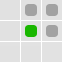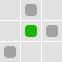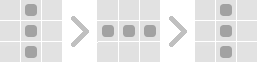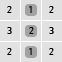Recently I have come across my old implementation of Conway’s Game Of Life in JavaScript I have played with a few years ago, had a long look and I didn’t like it.
At all.

## Conway’s Game Of Life

In short, it’s a cellular automaton, which means that it’s a matrix of cells where they live or die based on the surroundings to produce the next generation.

The state of each cell for the next generation is based on simple rules – three surrounding cells bring life, two cells will maintain the current state, and any other number of surrounding cells will result in death.Here is an example when there will be one more living cell (green) in the next generation. Since all others have two living neighbors, they will remain alive as well. It will stagnate after that – no new ones alive, no deaths.This one will result in just one living cell in the second generation, and there will be none after that.There are also some specific configurations, such as oscillators, for example this one.Here’s an image where each cell is marked with the number of surrounding cells alive, so it might be easier to grok.

I hope this gives you an idea… Have a look at Wikipedia for more detailed information. But let’s move on.

## The code

But the code was the true problem, so I decided to redesign it, just for the fun of it, and I thought it could be interesting to share this – the demos, a bit about the code and the redesign process.

The demos basically do the same thing, and the new one is not much to look at (visually), but this article is about the code redesign process and decisions involved. There will be a new article soon on the subject of visual redesign, so keep a lookout.

View the initial demo

View the new demo

## The old

Here’s the full source code of the old version if you like to have a look, but I’ll overview it piece by piece, so you don’t need to remember anything…

```
function Cell(i, j) {
this.Alive = false;
var el;
var x = j;
var y = i;
var id = "cell"+ i.toString() + j.toString();
this.Live = function() {
this.Alive = true;
el.className = el.className.replace("false", "true");
}
this.Die = function() {
this.Alive = false;
el.className = el.className.replace("true", "false");
}
this.ChangeState = function () {
var tmp = this.Alive.toString()
this.Alive = ! this.Alive;
el.className = el.className.replace(tmp, this.Alive.toString());
}
this.Red = function(OnOff) {
el.className = (OnOff) ? el.className+= " red" : el.className.replace(" red", "");
}
this.Green = function(OnOff) {
el.className = (OnOff) ? el.className+= " green" : el.className.replace(" green", "");
}
this.Element = function (parent) {
el = document.createElement("div");
el.onclick = function()
{
parent.ChangeCellState(x, y);
}
el.id = id;
el.className = "cell "+ this.Alive.toString();
return el;
}
}

function GameOfLife(Size, HTMLElementId) {
var Size = Size;
var Cells = new Array(Size);
var Next = new Array(Size);
var id = HTMLElementId;
var i, j;
var el = document.getElementById(id);
el.style.width = (5*Size) + "px";
for(i=0; i&amp;lt;Size; i++) {
Cells[i] = new Array(Size);
Next[i] = new Array(Size);
for(j=0; j&amp;lt;Size; j++) {
Cells[i][j] = new Cell(i, j);
el.appendChild( Cells[i][j].Element(this) );
}
}

this.ChangeCellState = function(x, y) {
Cells[y][x].ChangeState();
}

this.RandomStart = function() {
var OneOrZero = 0;
var i, j;
for(i=0; i&amp;lt;Size; i++) {
for(j=0; j&amp;lt;Size; j++) {
OneOrZero = Math.round(Math.round(Math.random() * 2)/2);
if (OneOrZero) Cells[i][j].ChangeState();
}
}
}

this.NextStep = function () {
var i, j;
for(i=0; i&amp;lt;Size; i++) {
for(j=0; j&amp;lt;Size; j++) {
var neighboursAlive = NeighboursAlive(i, j);
switch ( neighboursAlive ) {
case 3 : {
Next[i][j] = true;
break;
}
case 2 : {
Next[i][j] = (Cells[i][j].Alive) ? true : false;
break;
}
default : {
Next[i][j] = false;
}
}
}
}
for(i=0; i&amp;lt;Size; i++)
for(j=0; j&amp;lt;Size; j++)
(Next[i][j]) ? Cells[i][j].Live() : Cells[i][j].Die();
}

function NeighboursAlive(y, x) {
var horizontal = new Array( (x&amp;lt;=0) ? Size-1 : x-1, x , (x&amp;gt;=Size-1) ? 0 : x+1 );
var vertical = new Array( (y&amp;lt;=0) ? Size-1 : y-1, y, (y&amp;gt;=Size-1) ? 0 : y+1 );
var sum = 0;
var i, j;
for (i=0; i&amp;lt;3; i++)
for (j=0; j&amp;lt;3; j++)
if( vertical[i]!=y || horizontal[j]!=x ) sum += (Cells[ vertical[i] ][ horizontal[j] ].Alive) ? 1 : 0;
return sum;
}

var playerId;
var playing = false;
this.Play = function (name) {
if (!playing) {
this.NextStep();
playerId = setInterval( name +".NextStep()", 100);
playing = true;
}
}
this.Stop = function () {
if (playing) clearInterval(playerId);
playing = false;
}

}

It’s old school, no selectors, direct HTML elements injection and so on. Looks fine. But not the code.
Redesign
Ok, let’s start with the first entity, the cell. Here we go…
Cell class
Here is the original Cell class source code.
function Cell(i, j) {
this.Alive = false;
var el;
var x = j;
var y = i;
var id = "cell"+ i.toString() + j.toString();
this.Live = function() {
this.Alive = true;
el.className = el.className.replace("false", "true");
}
this.Die = function() {
this.Alive = false;
el.className = el.className.replace("true", "false");
}
this.ChangeState = function () {
var tmp = this.Alive.toString()
this.Alive = ! this.Alive;
el.className = el.className.replace(tmp, this.Alive.toString());
}
this.Red = function(OnOff) {
el.className = (OnOff) ? el.className+= " red" : el.className.replace(" red", "");
}
this.Green = function(OnOff) {
el.className = (OnOff) ? el.className+= " green" : el.className.replace(" green", "");
}
this.Element = function (parent) {
el = document.createElement("div");
el.onclick = function()
{
parent.ChangeCellState(x, y);
}
el.id = id;
el.className = "cell "+ this.Alive.toString();
return el;
}
}

The first thing that bothered me is that the Cell has a constructor with coordinates. The only place where the Cell uses the coordinate is to let the parent know of the state change, but this is closely related to the next eyesore.
The Cell knows about the Element that displays it and furthermore – operates on it, creating the representation and collecting events. While this can be regarded as convenient, it breaks the single responsibility principle, since we have a logical Cell model, but also it’s dealing with HTML. This means that it’s tightly coupled with representation.
So here’s the redesign.
gameOfLife.cell = function() {
return {
alive : false,
live : function() { this.alive = true; },
die : function() { this.alive = false; },
touch : function () { this.alive = ! this.alive; }
};
};

Much better, right? :)
GameOfLife class
How about the GameOfLife class…
function GameOfLife(Size, HTMLElementId) {
var Size = Size;
var Cells = new Array(Size);
var Next = new Array(Size);
var id = HTMLElementId;
var i, j;
var el = document.getElementById(id);
el.style.width = (5*Size) + "px";
for(i=0; i&amp;lt;Size; i++) {
Cells[i] = new Array(Size);
Next[i] = new Array(Size);
for(j=0; j&amp;lt;Size; j++) {
Cells[i][j] = new Cell(i, j);
el.appendChild( Cells[i][j].Element(this) );
}
}

this.ChangeCellState = function(x, y) {
Cells[y][x].ChangeState();
}

this.RandomStart = function() {
var OneOrZero = 0;
var i, j;
for(i=0; i&amp;lt;Size; i++) {
for(j=0; j&amp;lt;Size; j++) {
OneOrZero = Math.round(Math.round(Math.random() * 2)/2);
if (OneOrZero) Cells[i][j].ChangeState();
}
}
}

this.NextStep = function () {
var i, j;
for(i=0; i&amp;lt;Size; i++) {
for(j=0; j&amp;lt;Size; j++) {
var neighboursAlive = NeighboursAlive(i, j);
switch ( neighboursAlive ) {
case 3 : {
Next[i][j] = true;
break;
}
case 2 : {
Next[i][j] = (Cells[i][j].Alive) ? true : false;
break;
}
default : {
Next[i][j] = false;
}
}
}
}
for(i=0; i&amp;lt;Size; i++)
for(j=0; j&amp;lt;Size; j++)
(Next[i][j]) ? Cells[i][j].Live() : Cells[i][j].Die();
}

function NeighboursAlive(y, x) {
var horizontal = new Array( (x&amp;lt;=0) ? Size-1 : x-1, x , (x&amp;gt;=Size-1) ? 0 : x+1 );
var vertical = new Array( (y&amp;lt;=0) ? Size-1 : y-1, y, (y&amp;gt;=Size-1) ? 0 : y+1 );
var sum = 0;
var i, j;
for (i=0; i&amp;lt;3; i++)
for (j=0; j&amp;lt;3; j++)
if( vertical[i]!=y || horizontal[j]!=x ) sum += (Cells[ vertical[i] ][ horizontal[j] ].Alive) ? 1 : 0;
return sum;
}

var playerId;
var playing = false;
this.Play = function (name) {
if (!playing) {
this.NextStep();
playerId = setInterval( name +".NextStep()", 100);
playing = true;
}
}
this.Stop = function () {
if (playing) clearInterval(playerId);
playing = false;
}

}

So the GameOfLife class has really a lot of things going on, let’s have a look at all the things it does.

Has the Cells (the current generation) and Next matrix (the next generation of cells),
sets up both
changes the state of each cell
finds the neighbors of a cell
calculates the the next generation
randomly sets up the Cells matrix
plays and stops itself.

Whew.
Again, coupling with representation?! Seems that I really LOVED doing that back then…
Aside from that, I don’t like how it has the Play and Stop methods, I don’t think this belongs to this class, so I will extract it to a player a bit later. So here’s the redesign.
gameOfLife.game = function(size) {
var next = new Array(size);
var iterations = 0;

function neighboursAlive(x, y) {
var horizontalIndexes = getNeighbourIndexes(x);
var verticalIndexes = getNeighbourIndexes(y);
var sum = 0;
for (var i = 0; i &amp;lt; 3; i++) {
for (var j = 0; j &amp;lt; 3; j++) {
if (verticalIndexes[i]!=y || horizontalIndexes[j]!=x) sum += (_.cells[horizontalIndexes[i]][verticalIndexes[j]].alive) ? 1 : 0;
}
}
return sum;
};

function getNeighbourIndexes(x) {
return new Array( (x = size - 1) ? 0 : x + 1 );
};

var _ = {
size : size,
cells : new Array(size),
liveRule : 3,
noChangeRule : 2,

touch : function(x, y) {
cells[x][y].touch();
},

nextStep : function () {
var i, j;
for(i=0; i &amp;lt; size; i++) {
for(j=0; j &amp;lt; size; j++) {
var aliveInTheHood = neighboursAlive(i, j);
switch (aliveInTheHood) {
case _.liveRule : { next[i][j] = true; break; }
case _.noChangeRule : {
next[i][j] = (_.cells[i][j].alive) ? true : false;
break;
}
default : {
next[i][j] = false;
}
}
}
}
for(i=0; i &amp;lt; _.size; i++)
for(j=0; j &amp;lt; _.size; j++)
(next[i][j]) ? _.cells[i][j].live() : _.cells[i][j].die();
}
};

for(var i = 0; i &amp;lt; size; i++) {
_.cells[i] = new Array(size);
next[i] = new Array(size);
for(var j = 0; j &amp;lt; size; j++)
_.cells[i][j] = new gameOfLife.cell();
};

return _;
};

While I would say that this is much better, after some thought I still didn’t like it.
First of all, I don’t like all this running around the matrix checking states, calculating next state. Looks too convoluted.Second thing can be seen as a philosophical issue too – I really hate that the game is the divine force deciding the fate of cells. Looking from the software design side, it boils down to the fact that the game is dealing with the state of a cell based on the states of the surrounding cells. Too much cell-dealing stuff in the game object. So I took another go.

Round two
Cell
I have decided to move all the functionality that deals with the state of cells from the game to the cell class itself.
gameOfLife.cell = function () {
"use strict";
var future, _;
_ = {
alive : false,
touch : function () { _.alive = !_.alive; },
neighbours : [],
neighboursAlive : function () {
var sum = 0;
_.neighbours.forEach(function (neighbour) {
sum += +neighbour.alive;
});
return sum;
},
progress : function () {
if (future === undefined) {
_.futureIsBright();
}
_.alive = future;
future = undefined;
},
futureIsBright : function () {
if (future === undefined) {
var neighboursAlive = _.neighboursAlive();
future = neighboursAlive === gameOfLife.cell.neighboursToLive || (_.alive &amp;amp;&amp;amp; neighboursAlive === gameOfLife.cell.neighboursToStagnate);
}
return future;
}
};
return _;
};

gameOfLife.cell.neighboursToLive = 3;
gameOfLife.cell.neighboursToStagnate = 2;

So while the cell class now is nowhere clean as in the first round, it looks better.
The cell is now aware of its neighbors, talks to them, can see into the future and progress. :)
The neighbor’s changes awareness is possible due to the fact that the objects are passed by reference in JavaScript, so all changes that happen to neighbors will be visible because only the references are held, not the actual values.
Additionally, the game rules are now extracted to static variables on cell object.
To accommodate these changes, the game object has to change also, so the new version is below.
Game
gameOfLife.game = function (size) {
"use strict";
var _, i, j;

_ = {
cells : [],

touch : function (x, y) {
_.cells[x][y].touch();
},

progress : function () {
_.cells.forEach(function (row) {
row.forEach(function (cell) {
cell.futureIsBright();
});
});
_.cells.forEach(function (row) {
row.forEach(function (cell) {
cell.progress();
});
});
}
};

function correctIndex(x) {
return (x &amp;lt; 0) ? size - 1 : ((x &amp;gt; size - 1) ? 0 : x);
}

function neighbourIndexes(x) {
return [correctIndex(x - 1), x, correctIndex(x + 1)];
}

for (i = 0; i &amp;lt; size; i++) {
_.cells[i] = [];
for (j = 0; j &amp;lt; size; j++) {
_.cells[i][j] = new gameOfLife.cell();
}
}

_.cells.forEach(function (row, i) {
row.forEach(function (cell, j) {
neighbourIndexes(i).forEach(function (x) {
neighbourIndexes(j).forEach(function (y) {
cell.neighbours.push(_.cells[x][y]);
});
});
cell.neighbours.splice(4, 1);
});
});

return _;
};

The game now has only the cell matrix setup role and the access point to progress to next generation. Much better.
I played with the idea of using recursion to propagate setting the future state on all cells instead of running two loops in the propagate method, but I easily ran into an stack overflow, so sticking with the less elegant but practical solution.
Player
As I said before, I didn’t like how the game had play and stop methods, so the logic to run the game is now moved to the player object.
gameOfLife.player = function (game, screens) {
"use strict";
var i, playerId, refreshScreens, _;
for (i = 0; i &amp;lt; screens.length; i++) {
screens[i].setup(game);
}
refreshScreens = function () {
var i;
for (i = 0; i &amp;lt; screens.length; i++) {
screens[i].refresh();
}
};
_ = {
speed : 100,
play : function () {
playerId = window.setInterval(_.step, _.speed);
},
on : function () {
refreshScreens();
},
step : function () {
game.progress();
refreshScreens();
},
stop : function () {
if (playerId) {
window.clearInterval(playerId);
}
}
};
return _;
};

Simple stuff, allowing better modularity.
Screen
This is the concept that will enable different visual representations of the game. But it’s pretty simple for now.
gameOfLife.simpleScreen = function () {
"use strict";
var game, el;
return {
setup : function (game1, elementId) {
game = game1;
el = document.getElementById(elementId === undefined ? 'simpleRenderer' : elementId);
},
refresh : function () {
var i, j, html;
html = '';
for (i = 0; i &amp;lt; game.cells.length; i++) {
for (j = 0; j &amp;lt; game.cells[i].length; j++) {
html = html.concat(game.cells[i][j].alive ? '&amp;amp;diams;' : '.');
}
html = html.concat('&amp;lt;br/&amp;gt;');
}
el.innerHTML = html;
}
};
};

Random start
You may wander where did the random start method go. Since it doesn’t have anything to do with the game, but is just a fancy feature, I have extracted it to a separate function.
gameOfLife.randomize = function(game) {
var i, j;
for(i = 0; i &amp;lt; game.cells.length; i++) {
for(j = 0; j &amp;lt; game.cells.length; j++) {
if (Math.round(Math.round(Math.random() * 2) / 2))
game.cells[i][j].touch();
}
}
};

Done
At the end of this exercise I am pretty much satisfied with the outcome.
The redesign ended up looking like MVC pattern, where the model domain consists of cell and game classes, the player class is the controller, and the screen class is the view.
Here’s the demo, the tools and full code if you’re interested to have a look. In the next article I’ll work on the visual representation of the game, and I think it will be pretty interesting.
window.gameOfLife = {};

gameOfLife.cell = function () {
"use strict";
var future, askNeighboursFuture, _;

askNeighboursFuture = function () {
_.neighbours.forEach(function (neighbour) {
neighbour.futureIsBright();
});
};

_ = {
alive : false,
touch : function () { _.alive = !_.alive; },
neighbours : [],
neighboursAlive : function () {
var sum = 0;
_.neighbours.forEach(function (neighbour) {
sum += +neighbour.alive;
});
return sum;
},
progress : function () {
if (future === undefined) {
_.futureIsBright();
}
_.alive = future;
future = undefined;
},
futureIsBright : function () {
if (future === undefined) {
var neighboursAlive = _.neighboursAlive();
future = neighboursAlive === gameOfLife.cell.neighboursToLive || (_.alive &amp;amp;&amp;amp; neighboursAlive === gameOfLife.cell.neighboursToStagnate);
}

return future;
}
};

return _;
};

gameOfLife.cell.neighboursToLive = 3;
gameOfLife.cell.neighboursToStagnate = 2;

gameOfLife.game = function (size) {
"use strict";
var _, i, j;

_ = {
cells : [],

touch : function (x, y) {
_.cells[x][y].touch();
},

progress : function () {
_.cells.forEach(function (row) {
row.forEach(function (cell) {
cell.progress();
});
});
}
};

function neighbourIndexes(x) {
return [correctIndex(x - 1), x, correctIndex(x + 1)];
}

function correctIndex(x) {
return (x &amp;lt; 0) ? size - 1 : ((x &amp;gt; size - 1) ? 0 : x);
};

for (i = 0; i &amp;lt; size; i++) {
_.cells[i] = [];
for (j = 0; j &amp;lt; size; j++) {
_.cells[i][j] = new gameOfLife.cell();
}
}

_.cells.forEach(function (row, i) {
row.forEach(function (cell, j) {
neighbourIndexes(i).forEach(function (x) {
neighbourIndexes(j).forEach(function (y) {
cell.neighbours.push(_.cells[x][y]);
});
});
cell.neighbours.splice(4, 1);
});
});

return _;
};

gameOfLife.randomize = function (game) {
"use strict";
var i, j;
for (i = 0; i &amp;lt; game.cells.length; i++) {
for (j = 0; j &amp;lt; game.cells.length; j++) {
game.cells[i][j].alive = (Math.random() &amp;gt; 0.5);
}
}
};

gameOfLife.player = function (game, screens) {
"use strict";
var i, playerId, refreshScreens, _;
for (i = 0; i &amp;lt; screens.length; i++) {
screens[i].setup(game);
}
refreshScreens = function () {
var i;
for (i = 0; i &amp;lt; screens.length; i++) {
screens[i].refresh();
}
};
_ = {
speed : 100,
play : function () {
playerId = window.setInterval(_.step, _.speed);
},
on : function () {
refreshScreens();
},
step : function () {
game.progress();
refreshScreens();
},
stop : function () {
if (playerId) {
window.clearInterval(playerId);
}
}
};
return _;
};

gameOfLife.touchScreen = function () {
"use strict";
var game, el;
var _ = {
setup : function (game1, elementId) {
var i, j, dot;
game = game1;
el = document.getElementById(elementId === undefined ? 'simpleRenderer' : elementId);
for (i = 0; i &amp;lt; game.cells.length; i++) {
for (j = 0; j &amp;lt; game.cells[i].length; j++) {
dot = document.createElement('a');
dot.addEventListener('click', _.touch(i, j), false);
el.appendChild(dot);
dot = null;
}
el.appendChild(document.createElement('br'));
}
},
refresh : function () {
var i, j, dots, dot;
dots = el.getElementsByTagName('a');
for (i = 0; i &amp;lt; game.cells.length; i++) {
for (j = 0; j &amp;lt; game.cells[i].length; j++) {
dot = dots[j + i * game.cells.length];
dot.className = (game.cells[i][j].alive) ? 'cell alive' : 'cell dead';
}
}
},
touch : function(x, y) {
return function() { game.touch(x, y); _.refresh(); };
}
};
return _;
};

gameOfLife.simpleScreen = function () {
"use strict";
var game, el;
return {
setup : function (game1, elementId) {
game = game1;
el = document.getElementById(elementId === undefined ? 'simpleRenderer' : elementId);
},
refresh : function () {
var i, j, html;
html = '';
for (i = 0; i &amp;lt; game.cells.length; i++) {
for (j = 0; j &amp;lt; game.cells[i].length; j++) {
html = html.concat(game.cells[i][j].alive ? '&amp;amp;diams;' : '.');
}
html = html.concat('&amp;lt;br/&amp;gt;');
}
el.innerHTML = html;
}
};
};

function run() {
gameOfLife.spaceship = new gameOfLife.game(30);

gameOfLife.spaceship.cells.alive = true;
gameOfLife.spaceship.cells.alive = true;
gameOfLife.spaceship.cells.alive = true;
gameOfLife.spaceship.cells.alive = true;
gameOfLife.spaceship.cells.alive = true;

gameOfLife.station = new gameOfLife.player(gameOfLife.spaceship, [new gameOfLife.touchScreen()]);
gameOfLife.station.on();
}

View the new demo
The tools I have used:

JSLint, The JavaScript Code Quality Tool
QUnit, An easy-to-use JavaScript Unit Testing framework

1.